ISSN ONLINE(2319-8753)PRINT(2347-6710)

All submissions of the EM system will be redirected to Online Manuscript Submission System. Authors are requested to submit articles directly to Online Manuscript Submission System of respective journal.

# Solving Single Machine Scheduling Problem Using Type-2 Trapezoidal Fuzzy Numbers

 R. Helen1, R.Sumathi2 P.G. and Research, Department of Mathematics, Poompuhar College (Autonomous), Melaiyur, India P.G, Department of Mathematics, R.V.S.College of Engg. and Technology, Karaikal, Puducherry, India Related article at Pubmed, Scholar Google

Visit for more related articles at International Journal of Innovative Research in Science, Engineering and Technology

## Abstract

The objective of this paper is to find the single machine scheduling problem of n-jobs which minimizes the sum of the total tardiness of each job using dynamic programming method by type-2 trapezoidal fuzzy numbers. The effectiveness of the proposed method is illustrated by means of an example.

### Keywords

Machine Scheduling, Dynamic Programming Method, Type-2 Trapezoidal Fuzzy Numbers

### AMS Subject Classification

94D05, 90-XX , Aug 2010

### INTRODUCTION

The single machine scheduling problem with single processor (Machine) consists of single machine to process n-jobs. The jobs may be independent or dependent. An single machine scheduling problems can provide help and insight into resolving, understanding, managing and modeling more complex multi-machine scheduling problems. In this paper, we propose n-jobs to be processed on single machine scheduling problem (SMSP) involving type-2 trapezoidal fuzzy processing times and type-2 trapezoidal fuzzy due date. The different due date for each job be considered which meet the demand of customer with more satisfaction level. The main objective of this paper is to scheduling the job and minimizing the total tardiness. This method is become lucrative to make decision. In most of the real life problem, there are elements of uncertainty in process. In practical situation processing times and due date are not always deterministic. So, we have associated with fuzzy environment. Dynamic programming problem is the main concept of number of jobs is equal to number of stages.
The concept of a type-2 fuzzy set, which is an extension of the concept of an arbitrary fuzzy set, was introduced by Zadeh . A fuzzy set is two dimensional and a type-2 fuzzy set is three dimensional, type-2 fuzzy sets can better improve certain kinds of inference than do fuzzy sets with increasing imprecision, uncertainty and fuzziness in information. A type-2 fuzzy set is characterized by a membership function, i.e., the membership value for each element of this set is a fuzzy set in [0,1], unlike an ordinary fuzzy set where the membership value is a crisp number in [0,1].

### REVIEW OF LITERATURE

Various researchers have done a lot of work in different directions. Ishii and Tada  considered a single machine scheduling problem minimizing the maximum lateness of jobs with fuzzy precedence relations. Hong et.al.,  introduced a single machine scheduling problem with fuzzy due date. Itoh and Ishii  proposed a single machine scheduling problem dealing with fuzzy processing times and due date. Gawiejnowiez et.al., deals with a single machine time dependent scheduling problem. Lawler  applied a dynamic programming approach to the single machine total tardiness problem. Uzsoy and Velasquez  addressed the problem of scheduling a single machine subject to family dependent set-up times in order to minimize maximum lateness. Graham and Lawler  introduced on single machine scheduling problem with non-zero ready times. Emmons  studied the single machine scheduling problem to minimize the total tardiness. Ghorhanali Mohammad  introduced the concept of single machine and the processing times of the jobs in fuzzy environment.
The paper is organized as follows: In section 2, deals with the preliminaries. In section 3, arithmetic operations on type-2 trapezoidal fuzzy number and ranking function are discussed. In section 4, we introduced a brief note on single machine scheduling problem. In section 5, the effectiveness of the proposed method is illustrated by means of an example.

### PRELIMINARIES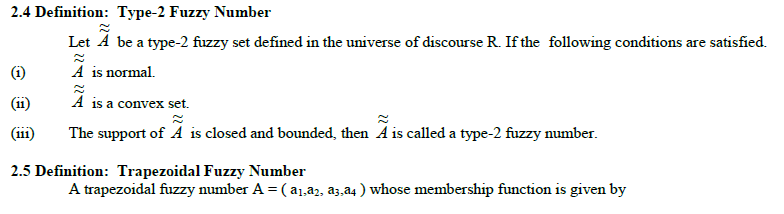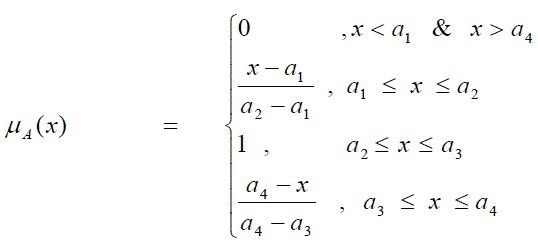Definition: Type-2 Trapezoidal Fuzzy Number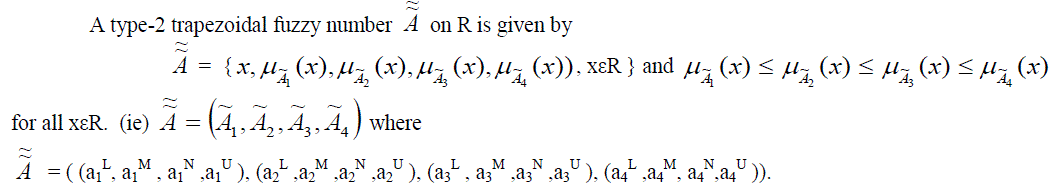### Arithmetic Operations on Type-2 Trapezoidal Fuzzy Numbers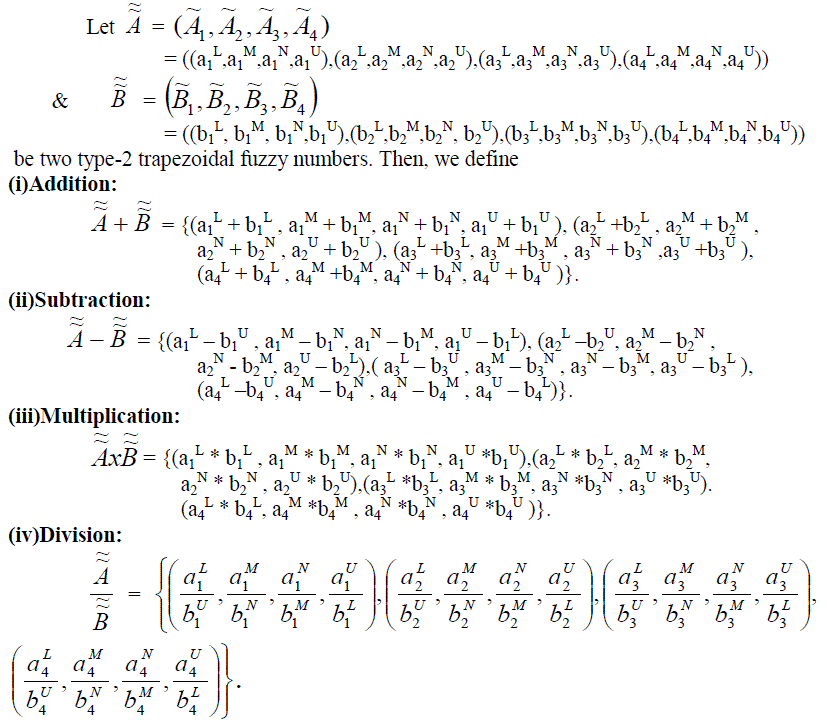#### Ranking on Type-2 Trapezoidal Fuzzy Number

Let F( R ) be the set of all type-2 normal trapezoidal fuzzy numbers. One convenient approach for solving numerical valued problem is based on the concept of comparison of fuzzy numbers by use of ranking function. An effective approach for ordering the elements of F ( R ) is to define a linear ranking function R: F ( R ) → R which maps each fuzzy number into R.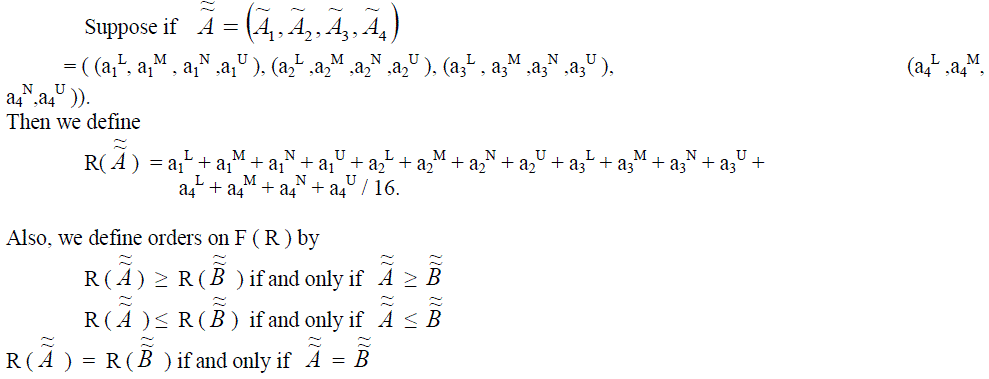### SINGLE MACHINE SCHEDULING PROBLEM

#### Tardiness:

Tardiness is the lateness of job j if it fails to meet its due date; otherwise, it is zero. It is defined as :Notations: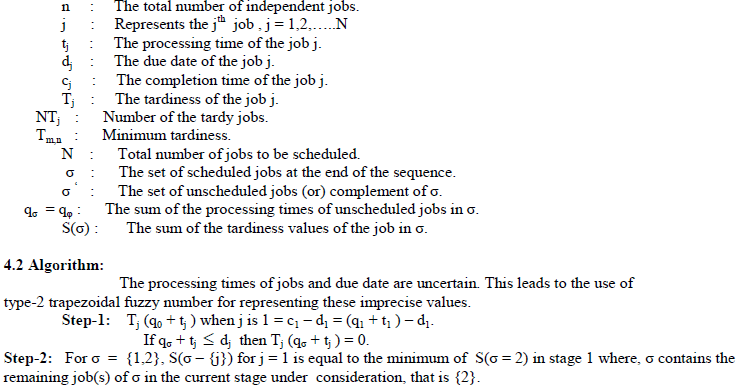Step-3: For σ = {1,2,3} in stage3, S(σ – {j}) for j = 1 is equal to the minimum of S(σ = 2,3) in stage2 where , σ contains the remaining jobs of σ in the current stage under consideration, that is {2,3}.
Step-4: For σ = { 1,2,3,5} in stage 4, S( σ – {j}) for j = 2 is equal to the minimum of S(σ = 1,3,5) in stage3 where, σ contains the remaining jobs of σ in the current stage under consideration, that is {1,3,5}.
Step-5: For σ = { 1,2,3,4,5} in stage 5, S(σ – {j}) for j = 5 is equal to the minimum of S(σ = 1,2,3,4) in stage 4 where, σ contains the remaining jobs of σ in the current stage under consideration, that is {1,2,3,4}.
NUMERICAL ILLUSTRATION
Consider a single machine scheduling problem in which each of the 5 jobs can be fully processed in the single machine. The type-2 trapezoidal fuzzy processing times and type-2 trapezoidal fuzzy due date for each jobs are given in the following table:
Table-1: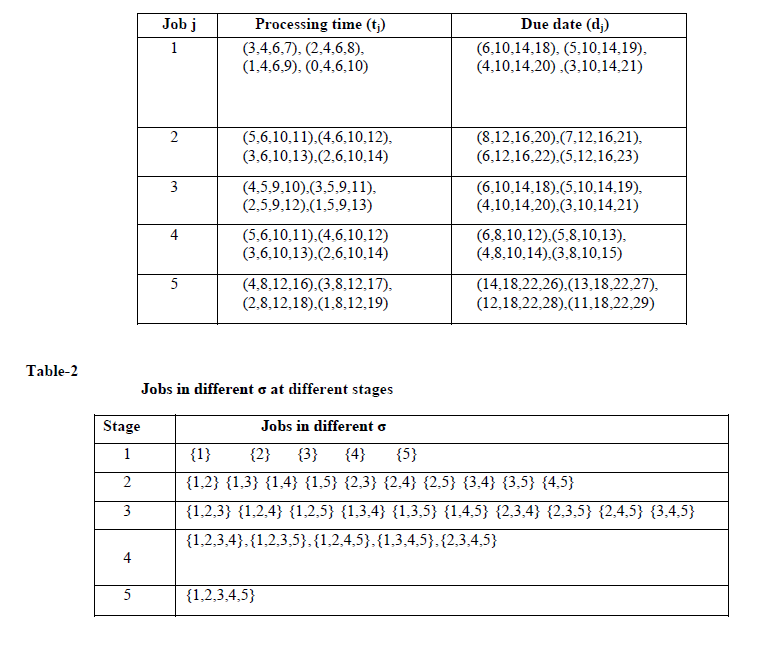Step-1: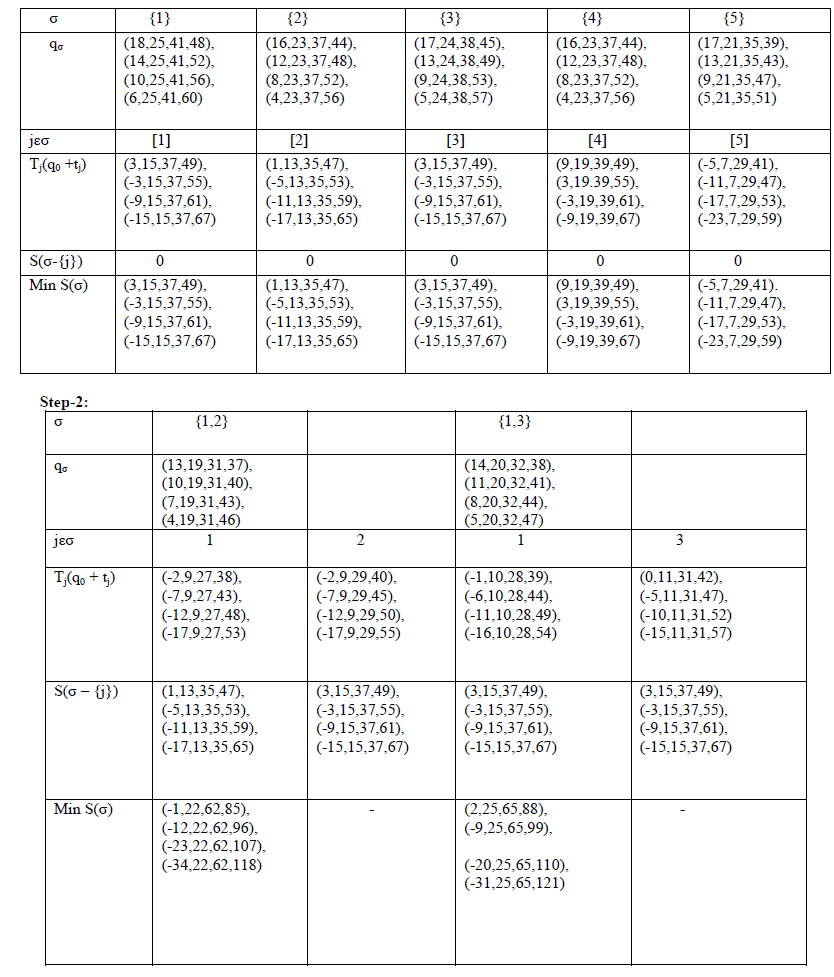Optimal Sequence of Jobs = 4 → 1 →3 → 2 → 5
Minimum Total Tardiness = 41.

### CONCLUSION

We considered a single machine scheduling problem (SMSP) with fuzzy processing times and fuzzy due date to minimize the total tardiness. This method is very easy to understand each stage that will help the decision maker in determining a best schedule for a given set of jobs effectively. This method has significant use of practical results in industries.

### References

. Emmons.H, “ One machine sequencing to minimize certain functions of Tradiness”, Operations Research,17,701-715,1969.

. Gawiejnowiez. S. et.al., “ Analysis of a time dependent scheduling problem by signature of Deterioration rate sequences”, Discrete Applied Mathematics, vol.154, 2150- 2166,2006

. Ghorhanali Mohammadi, “Single machine common flow allowance scheduling with a fuzzy rate-modifying activity”, Indian Journal of Science and Technology, vol.4, No.7,726-730,2011. .

. Graham.R.L, “ Bounds on multiprocessing timing anomalies, SIAM. Journal of Application Mathematics, 17,416-429,1969.

. Hong.et.al., “ Using fuzzy set theory in a scheduling problem”, A case study Fuzzy sets and systems, vol.2,153-165,1979.

. Ishii H and Tada M, “ Single machine scheduling problem with fuzzy precedence relation”,European Journal of Operational Research, vol.6, 141-147,2000.

. Itoh T. and Ishii H. “ Fuzzy due-date scheduling problem with fuzzy processing times”, International Transactions in Operational Research, vol.6, 639-647,2000

. Lawler E.L., Lenstra J.K.et.al., “Sequencing and Scheduling” , Algorithm and complexity in Handlooks in Operations Research and Management Science, vol.1, 1990.

. Lawler E.L., “ A Pseudopolynomial algorithm for sequencing jobs to minimize total tardiness”, Annals of Discrete Mathematics, 331- 342,1977.

. M.Mizumoto and K.Tanaka, “ Some properties of fuzzy sets of type-2”, Information and Control, vol.31,312-340,1976.

. J,M, Mendal F,Liu and D.Zhai, “a-plane representation for type-2 fuzzy sets: theory and applications”, IEEE Trans. Fuzzy Systems vol.17,1189-1207,2009.

. Q. Mendoza, P.Melin and G,Licea, “ A hybrid approach for image recognition combining type-2 fuzzy logic, modular neural networks and the sugeno integral”, Information Sciences, vol.179,2078-2101.

. Uzsoy R and Velasquez JD, “ Heuristics for minimizing maximum lateness on a single machine with family-dependent set up times”, Computers and Operations Research,35,2018-2033,2008.

. C.Wagner and H. Hagras, “ Towards general type-2 fuzzy logic systems based on zslices”, IEEE Trans. Fuzzy System s, vol.18, 637-660, 2010.

. Zadeh. J.A, “ The fuzzy concept of a Linguistic variable and its applications to approximate reasoning”, International Journal Inform Sciecne, 8,199-240,1997.